Concept 17: Price, Income and Cross-Price Elasticities of Demand | IFT World
101 Concepts for the Level I Exam

# Concept 17: Price, Income and Cross-Price Elasticities of DemandElasticity of demand is measured as a ratio of percentage change in quantity demanded to a percentage change in other variables.

Own-price elasticity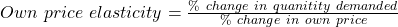• Own-price elasticity of demand is usually always negative.
• If |own price elasticity| > 1, then demand is elastic.
• If |own price elasticity| < 1, then demand is inelastic.
• If own price elasticity = -1, then demand is unit, or unitary, elastic.

Income elasticity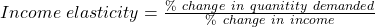• If income elasticity > 0, then the good is a normal good.
• If income elasticity < 0, then the good is an inferior good.

Cross price elasticity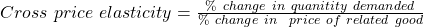• If cross price elasticity > 0, then the related good is a substitute.
• If cross price elasticity < 0, then the related good is a complement.

A demand function for chairs is as follows: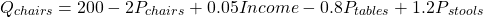At current average prices, a chair costs $50, a table costs$100 and a stool costs $30. Average income is$5,000. Calculate the income elasticity of demand for chairs.

Solution:

Substitute current values for the independent variables (except income)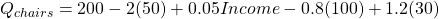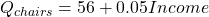The slope of income is 0.05

For an income of \$5,000; Qchairs = 306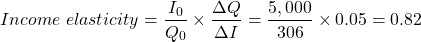Factors impacting the own price elasticity of demand for a product include:

• Substitutes: If the number of substitutes for this product is high, then elasticity will be high.
• Portion of total budget: If the portion of total budget spent on this product is high, then elasticity will be high.
• Time horizon: If the time horizon we consider is long, then elasticity will be high. This is because consumers will have enough time to respond to changes in the price of this product.
• Discretionary (optional) versus non-discretionary (necessary): If the product is discretionary rather than non-discretionary, then the elasticity will be high.

LIVE WEEKEND CLASSES *For 2024 Exam candidates*
This is default text for notification bar